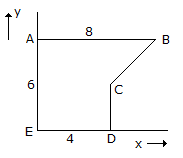# Civil Engineering - UPSC Civil Service Exam Questions

46.

Two perfectly elastic spheres of equal mass moving in the same direction with their velocities in the ratio 2 : 1 have an impact. After the impact, the two spheres will

 A. move in the same direction B. have in velocity in the ratio of 1 : 2 C. move in opposite directions with velocity the ratio of 1: 2 D. move in the same direction with velocity the ratio of 1: 2

Explanation:

No answer description available for this question. Let us discuss.

47.

For the design of a simply supported RCC T-beam, the ratio of the effective span to the overall depth of the beam should not exceed

 A. 10 B. 20 C. 30 D. 40

Explanation:

No answer description available for this question. Let us discuss.

48.

The typical density in kg/cm (in situ) of well-compacted municipal solid waste in landfill is in the range of

 A. 100 to 300 B. 310 to 500 C. 550 to 850 D. 900 to 1100

Explanation:

No answer description available for this question. Let us discuss.

49.

What is the y-coordinate of the centroid of the area ABCDE shown in the figure given aside ?A. 3.2 B. 3.3 C. 3.4 D. 3.5

Explanation:

No answer description available for this question. Let us discuss.

50.

In a plate load test on sandy soil, the test plate of 60 cm x 60 cm undergoes a settlement of 5 mm at a pressure of 12 x 104 N/m2. What will be the expected settlement of 3 m x 3 m footing under same pressure ?

 A. 25 mm B. 20 mm C. 15 mm D. 9 mm